### How to Use the Aggregate Function in Excel

The aggregate function is a very useful function in excel that can perform almost twenty different of tasks at ease.

It is a function that will return an aggregation in either database or list. It has the ability to ignore certain areas that the user has chosen.

### Aggregate Function’s Arguments

The aggregate function has a syntax that works together in providing the values that it returns with, which are in two different form, one is the reference form which has function number, options, references that goes from 1 to about 253 different ones. Another is the array form, which is the function number, followed by options, array and finally the k.

Function number (function_num): This is a required part of the argument, and there are between number 1 and 19 for the user to specify which function that he or she would like to use.

Options: This is another required argument, which is a numerical value that will determine which values are to be ignored in the evaluation range for functioning, which has between 0 or omitted to 7.

Reference 1 [ref1]: This is required, and the first argument that is of number for function that will take different arguments of number for which the user wishes to aggregate its value.

Reference 2+: [ref2]: An optional part of arguments that contains number, and extends from 2 to 253 different arguments for the user to use.

### A Simple Formula

This is the example that clarifies that it is possible to use the aggregation formula to find the average of various prices we have set for the products over the months. We are going to use the aggregation formula to find the result.### Aggregate Twice at the Same Time

The business has been going through some changes, and we needs to know how it looks like, so we could find the answers that we are looking for, and because we have changed the prices so much we needs to know how much we have made. The formulas will make it possible to find out how much we have made in the business, which is informed through sales, and with all the dynamic changes we have made to the price setting of the same product.### Using Aggregation to Solve a Mystery Part 1

There is a little mystery that we are trying to solve. We needs to find a simple resource that will explain the answers that we are looking for, which is a simply about verifying that the earnings is truly the amount of money we have made in earnings. This is to be clarify that we need to know the minimum amount of money that we will be making if we sell all the products with the minimum amount of product’s price.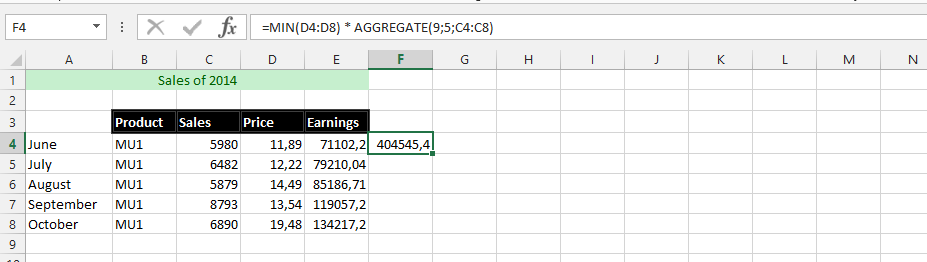### Using Aggregation to Solve a Mystery Part 2

This example is about making it possible to know the maximum amount of money we make if we have sold all the products with highest price rate. This is where the formulas would be different from the information in the previous example.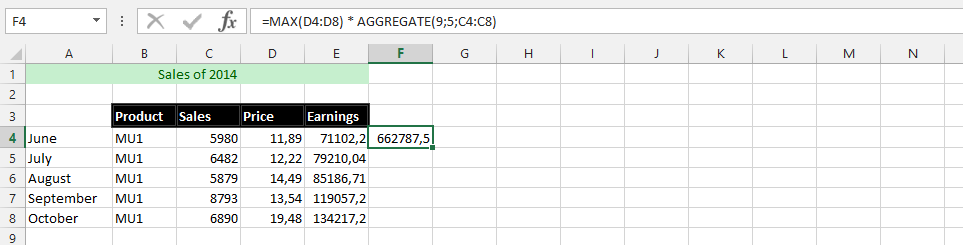### Aggregation Solving a Mystery Part 3

This is about finding out how the average performance is with the aggregation formula, which means using the average formula with the aggregation formula, to find out how much we have made in average.### Creativity with Aggregation Formula

This example is going to provide the possibilities of finding an appropriate and correct answer, which make it possible and easy to know how the average performance would be affecting the whole performance of the business. This is where it is necessary to know what is best for the company, because it makes it easy to make different assessment.### VLOOKUP Usage with AGGREGATION

This example is using the combination of Vlookup with aggregation formula together for the successfulness of finding a specific answer. This is why we are going to use both formulas in one single formula to find answer.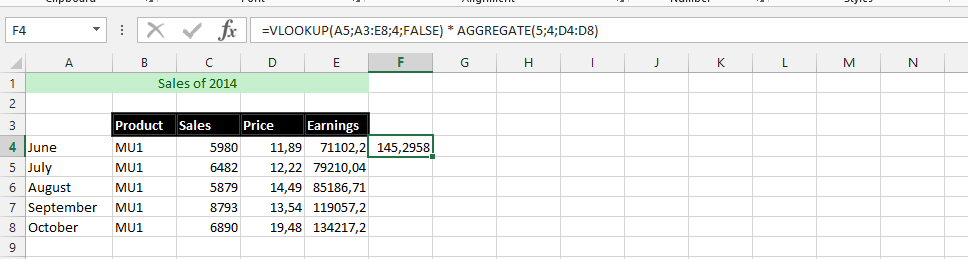### Two Aggregate to Divide

We are using two aggregation formula, but this time there is a divide between them. We are going to use this to find answers with the information, but we have labeled all the data with texts, which is what we are going to use to find the answers we are looking for, which the picture below provide.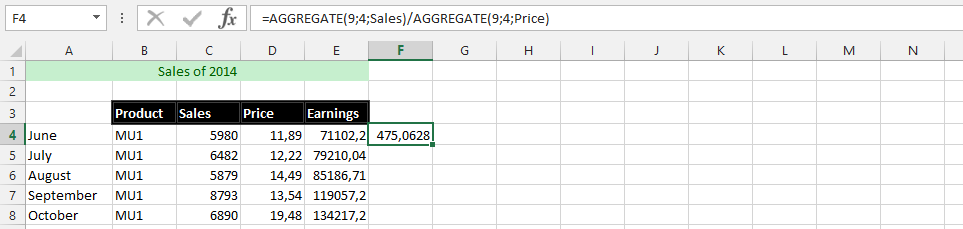### SUM and AGGREGATION

This is the example, where we are using both the sum and aggregation to find the total value that we will be using when the whole information is very clear. We want to use both the sum and aggregation function to get how much we could make from the information.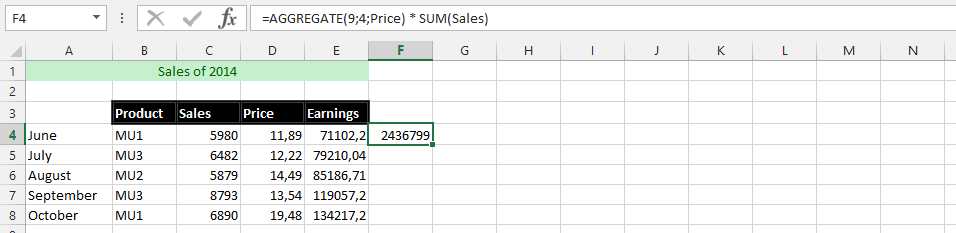### Counting with Aggregation

This is a formula that has purposely been to find out how many sales data are available, and it is not about knowing how the data has been assessed. This is where both count and aggregate formula would be very useful for the successfulness of finding answer.## Template

```Further reading: